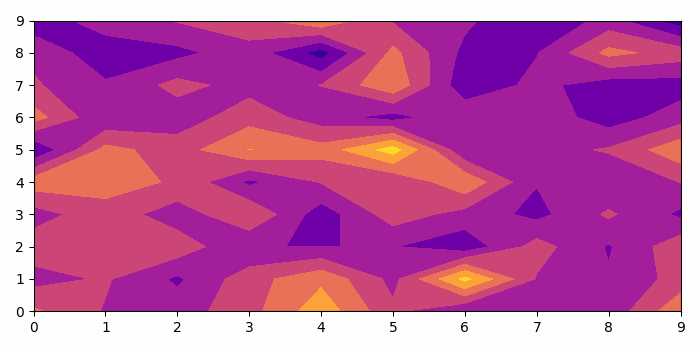# Animation with contours in matplotlib

To animate with contours in matplotlib, we can take the following steps

## Steps

• Set the figure size and adjust the padding between and around the subplots.

• Create data for the contour plot.

• Create a figure and a set of subplots.

• Generate an animation by repeatedly calling a function *animate* where the animate() method changes the contour data points.

• To display the figure, use Show() method.

## Example

import numpy as np
import matplotlib.pyplot as plt
import matplotlib.animation as animation

# Set the figure size
plt.rcParams["figure.figsize"] = [7.00, 3.50]
plt.rcParams["figure.autolayout"] = True

# Random data for the contour plot
data = np.random.randn(800).reshape(10, 10, 8)

# Create a figure and a set of subplots
fig, ax = plt.subplots()

# Method to change the contour data points
def animate(i):
ax.clear()
ax.contourf(data[:, :, i], cmap='plasma')

# Call animate method
ani = animation.FuncAnimation(fig, animate, 5, interval=50, blit=False)

# Display the plot
plt.show()

## Output

It will produce the following output −